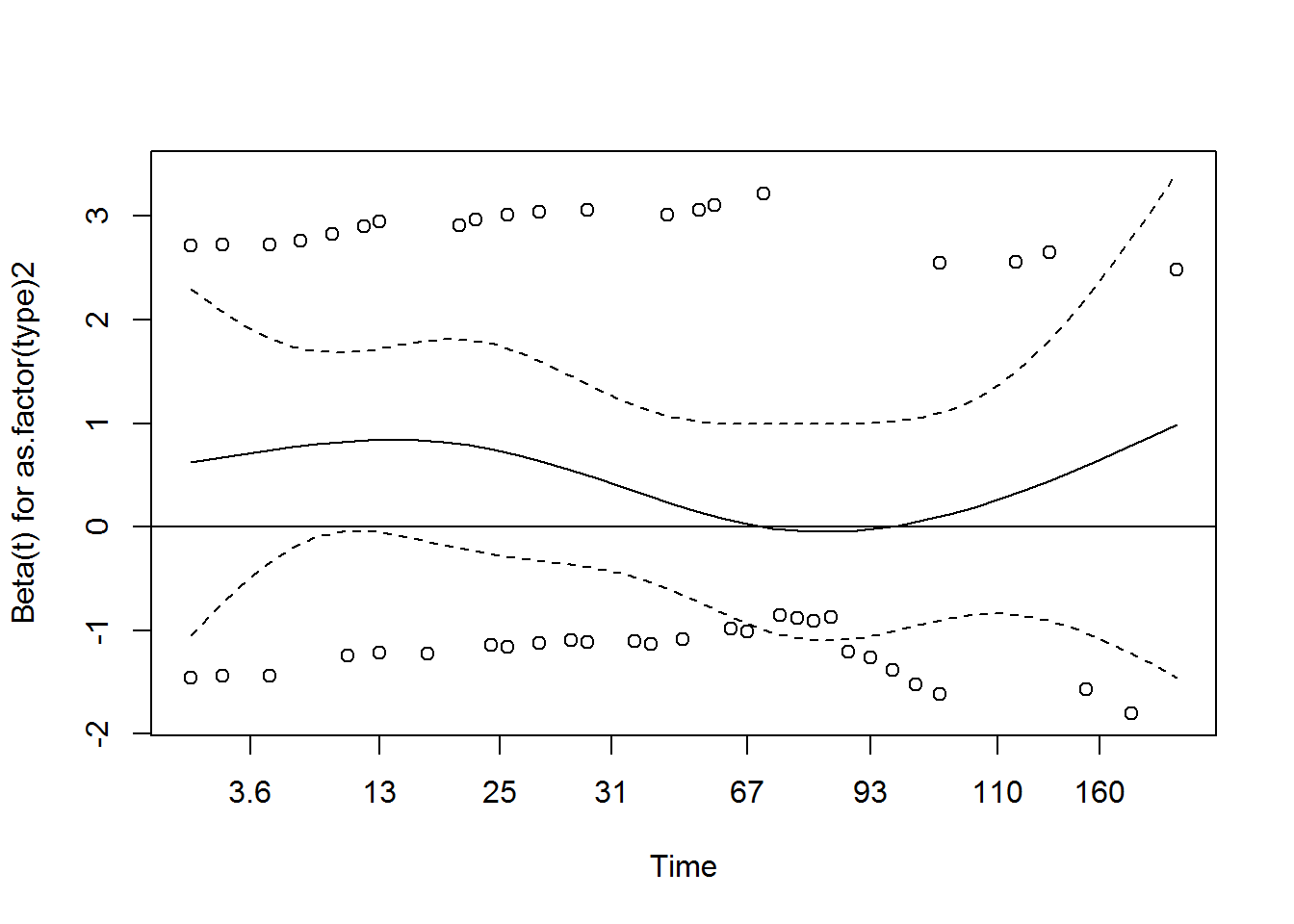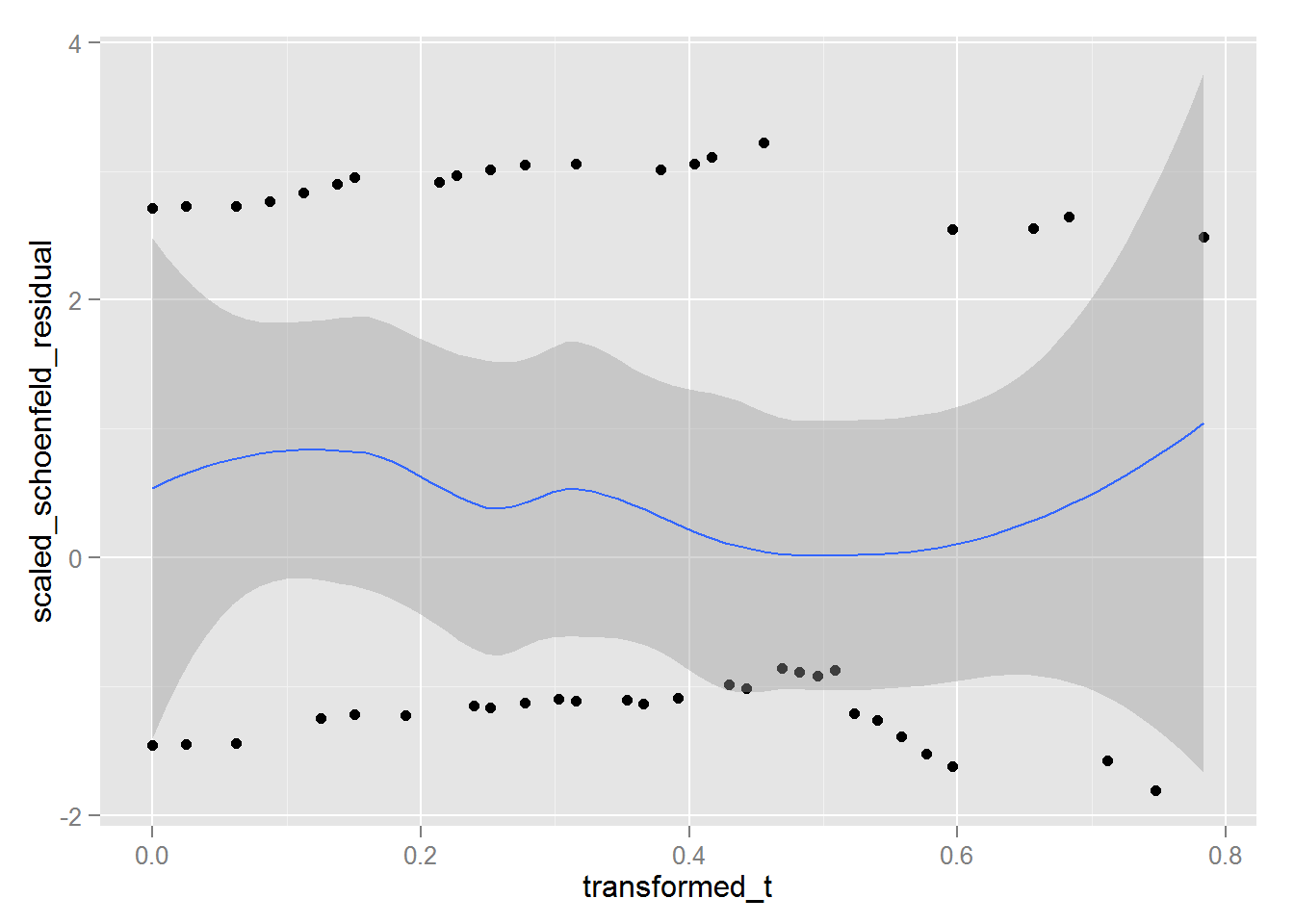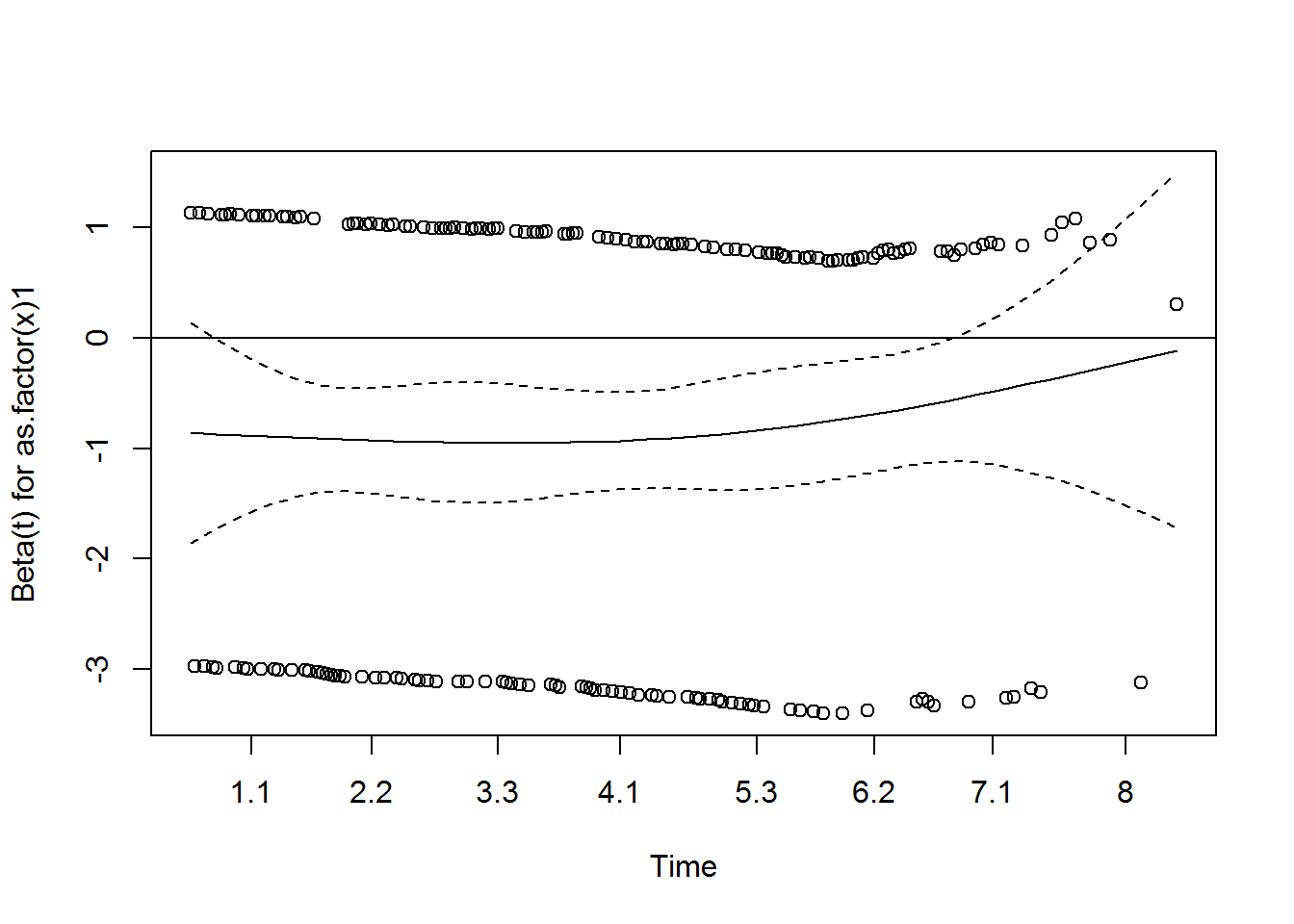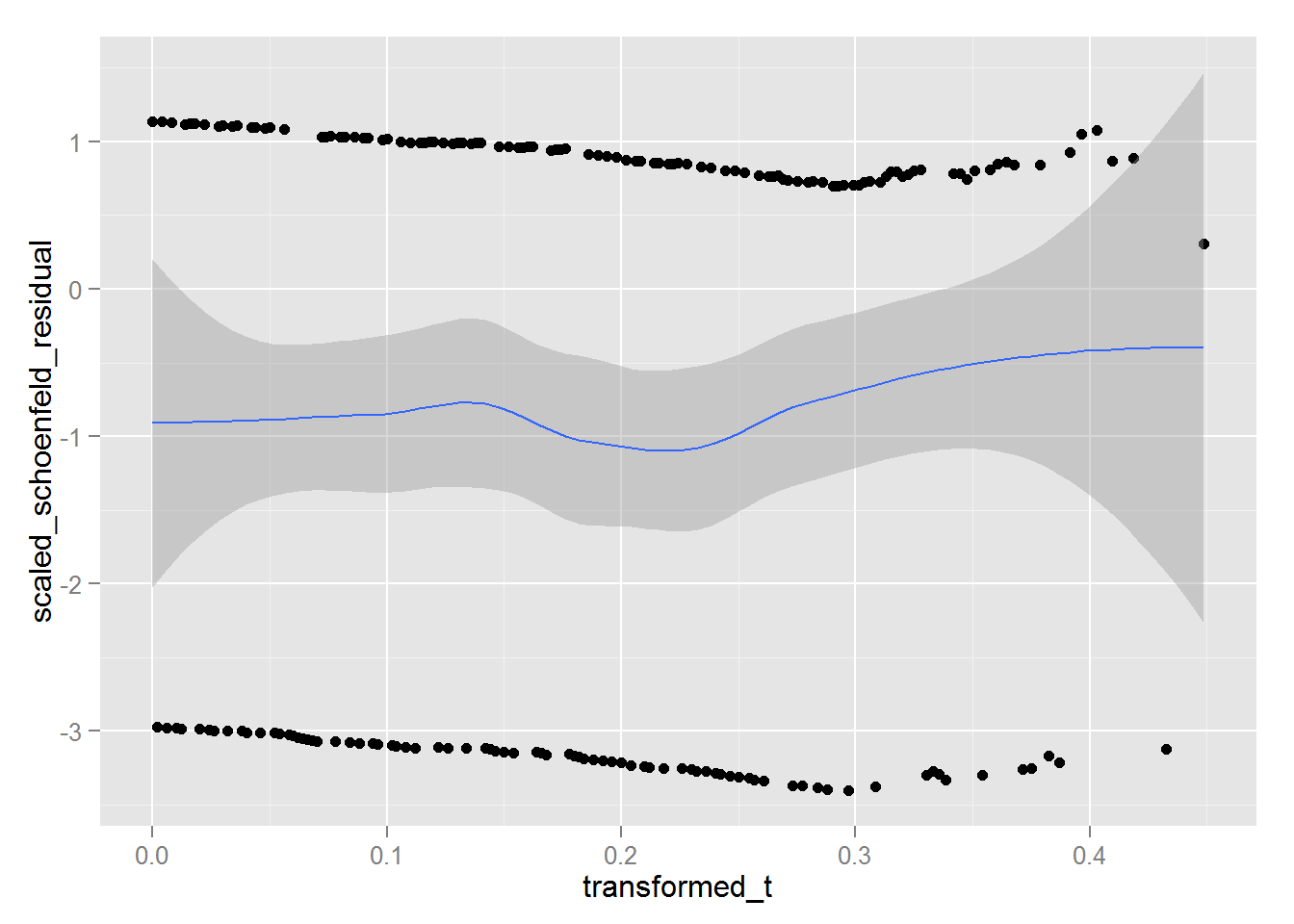## Objective:

1. Find out effect of covariates on hazard ratio without making any assumption about the shape of the hazard function.
2. Interpret the model outputs. Evaluate model summary, coefficient significance.
3. Validate proportional hazards assumption.

## Solution:

This can be done using Cox Propotional Hazard model. (No time-varying covariates)

survival package has coxph function to accomplish this.

1. Surv function to get the data in the right format, incorporating censoring information.
2. which will then be passed to coxph function alongwith the covariate(s) to find out their effect.
3. cox.zph to validate proportional hazard assumption.

## Code:

library(magrittr)
{
library(OIsurv)
library(dplyr)
library(broom)
library(ggplot2)
library(survsim)
}
# %>%
#   suppressMessages()
# Real dataset from KMsurv package
data(tongue)
# summary(tongue)
# help(tongue)

This dataset is about 80 male subjects who were diagnosed with one of the 2 types of cancer of the tongue. Time tp death is expressed in weeks. More info on this can be found from the package documentation for this dataset using help(tongue).

Shown below is coxph model with cancer type as covariate.

# COX PH
tongue_coxph = coxph(Surv(time = time, event = delta) ~ as.factor(type), data = tongue)
tongue_coxph_tidy = tidy(tongue_coxph)
tongue_coxph_tidy # equivalent of print()
##               term  estimate std.error statistic    p.value    conf.low
## 1 as.factor(type)2 0.4663742 0.2804476  1.662964 0.09631971 -0.08329303
##   conf.high
## 1  1.016041
• The confidence interval for the coefficient estimate [-0.083293, 1.0160414] contains 0, implies the relationship is not significant.

• The likelihood ratio test against the null model (below) has p-value of 0.1020272 which again supports this.

glance(tongue_coxph) # equivalent of summary()
##    n nevent statistic.log p.value.log statistic.sc p.value.sc
## 1 80     53      2.673567   0.1020272     2.814338 0.09342544
##   statistic.wald p.value.wald  r.squared r.squared.max concordance
## 1           2.77   0.09631971 0.03286732     0.9929755   0.5642941
##   std.error.concordance    logLik     AIC  BIC
## 1            0.03561883 -196.9975 395.995 -Inf
# plot(survfit(tongue_coxph, newdata = as.data.frame(cbind(type = 1)))) # for prediction, only the inputs/covariates need to be provided

Now, even though the coefficient is not significant, we can test and see if the model assumptions are satisfied.

• The proportional hazards assumption essentially means the coefficient remains constant over time. So, if we can find correlation between time and the coefficient and test if that’s significant or not, we’ll able to test the proportional hazards assumption.
• To test this assumption we can use cox.zph function. It tests correlation between Schoenfeld residuals and (transformed) time using a chi-square test. For this test, p-value less than certain threshold (say 0.5) would imply the correlation between the residuals and (transformed) time is significant and proportional hazards assumption does not hold. (More info on schoenfeld residual)
# Validating Cox PH Assumptions
validate_coxph = cox.zph(tongue_coxph, transform = "km")
validate_coxph
##                      rho chisq     p
## as.factor(type)2 -0.0958 0.473 0.491
• From the low p-value, the proportional hazards assumption does not seem to hold here.

• The plot below shows the scaled Schoenfeld residuals. Ideally for PH assumption to hold, this should be a flat straight line. (Like any other residuals, these residuals should exhibit random pattern. )

plot(validate_coxph)
abline(h=0)validate_gg = data.frame(cbind(x = validate_coxph[["x"]],
y = validate_coxph[["y"]]))
## Warning in data.row.names(row.names, rowsi, i): some row.names duplicated:
## 2,4,5,7,9,14,15,17,22,24,27,28,47,48 --> row.names NOT used
names(validate_gg) = c("transformed_t", "scaled_schoenfeld_residual")

ggplot(data = validate_gg, aes(x = transformed_t, y = scaled_schoenfeld_residual)) +
geom_point() +
geom_smooth(method = "loess")### Simulating a dataset using simple.surv.sim function from survsim package.

(The same dataset was used in prior post)

• Time-to-event: Assumed to be following weibull distribution with p = 1.5 i.e. the mode of the time to event is closer to median then for exponential distribution (p = 1). Exponential distribution has it’s mode at 0, as we increase p, the mode moves to the right.
• Time-to-censoring: Assumed to be following weibull distribution with p = 10 i.e. censoring is happening mostly in the latter part of the survival curve.
• Covariate: 1 Binary covariate with p = .7 and beta of 0.6. (In the following post I’ll try to reverse-engineer this beta using coxph or other similar models)

Following is the same analysis using this simulated dataset. Try and see if you can make sense of code and the output.

set.seed(2365)
d1 = simple.surv.sim(# event dist - weibull with p = 1.5
n = 500, foltime = 1000, dist.ev = 'weibull', anc.ev = 1.5, beta0.ev = 2,
# censoring dist - weibull with p = 10
dist.cens = 'weibull', anc.cens = 10, beta0.cens = 2.01,
# 1 binary covariate
beta = list(c(.6)), x = list(c("bern", .7))
)
# View(d1)

# summary(d1)
summary(as.data.frame(d1))
##       nid            status          start        stop              z
##  Min.   :  1.0   Min.   :0.000   Min.   :0   Min.   :0.1452   Min.   :1
##  1st Qu.:125.8   1st Qu.:0.000   1st Qu.:0   1st Qu.:4.8752   1st Qu.:1
##  Median :250.5   Median :0.000   Median :0   Median :6.6124   Median :1
##  Mean   :250.5   Mean   :0.372   Mean   :0   Mean   :5.9297   Mean   :1
##  3rd Qu.:375.2   3rd Qu.:1.000   3rd Qu.:0   3rd Qu.:7.4102   3rd Qu.:1
##  Max.   :500.0   Max.   :1.000   Max.   :0   Max.   :9.1243   Max.   :1
##        x
##  Min.   :0.000
##  1st Qu.:0.000
##  Median :1.000
##  Mean   :0.714
##  3rd Qu.:1.000
##  Max.   :1.000
# COX PH
d1_coxph = coxph(Surv(time = start, time2 = stop, event = status) ~ as.factor(x), data = d1)
d1_coxph_tidy = tidy(d1_coxph)
d1_coxph_tidy # equivalent of print()
##            term   estimate std.error statistic      p.value  conf.low
## 1 as.factor(x)1 -0.8220694 0.1486678 -5.529572 3.210132e-08 -1.113453
##    conf.high
## 1 -0.5306858
glance(d1_coxph) # equivalent of summary()
##     n nevent statistic.log  p.value.log statistic.sc   p.value.sc
## 1 500    186      28.63993 8.716611e-08     32.31914 1.308183e-08
##   statistic.wald p.value.wald  r.squared r.squared.max concordance
## 1          30.58 3.210132e-08 0.05567025     0.9871835   0.5959589
##   std.error.concordance    logLik     AIC  BIC
## 1            0.01658598 -1074.935 2151.87 -Inf
# plot(survfit(d1_coxph, newdata = as.data.frame(cbind(type = 1)))) # for prediction, only the inputs/covariates need to be provided

The coefficient for covariate x is significant but is -0.8220694, quiet different from the parameter we used to simulate this data i.e. 0.6. This is happening partly due to the fact that for coxph we are finding the coefficients using partial likelihood without making any assumptions around the time-to-event distribution.

# Validating Cox PH Assumptions
validate_d1_coxph = cox.zph(d1_coxph, transform = "km")
validate_d1_coxph
##                  rho chisq     p
## as.factor(x)1 0.0659 0.798 0.372

From not so low p-value (), the proportional hazards assumption holds here.

The scaled Schoenfeld residuals plot below also looks flat for the most part, seems to be increasing for higher values of time.

plot(validate_d1_coxph)
abline(h=0)Below is a ggplot2 version using loess fit telling the same story.

validate_d1_gg = data.frame(cbind(x = validate_d1_coxph[["x"]],
y = validate_d1_coxph[["y"]]))
names(validate_d1_gg) = c("transformed_t", "scaled_schoenfeld_residual")

ggplot(data = validate_d1_gg, aes(x = transformed_t, y = scaled_schoenfeld_residual)) +
geom_point() +
geom_smooth(method = "loess")